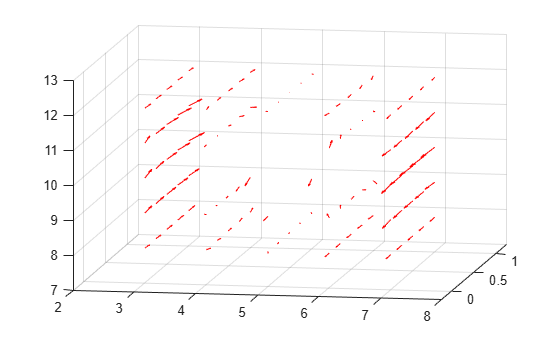interpolateMagneticFlux

Interpolate magnetic flux density in magnetostatic result at arbitrary spatial locations

Description

example

Bintrp = interpolateMagneticFlux(magnetostaticresults,xq,yq) returns the interpolated magnetic flux density at the 2-D points specified in xq and yq.

example

Bintrp = interpolateMagneticFlux(magnetostaticresults,xq,yq,zq) uses 3-D points specified in xq, yq, and zq.

example

Bintrp = interpolateMagneticFlux(magnetostaticresults,querypoints) returns the interpolated magnetic flux density at the points specified in querypoints.

Examples

collapse all

Create an electromagnetic model for magnetostatic analysis.

emagmodel = createpde('electromagnetic','magnetostatic');

Create a square geometry and include it in the model. Plot the geometry with the edge labels.

R1 = [3,4,-1,1,1,-1,1,1,-1,-1]';
g = decsg(R1,'R1',('R1')');
geometryFromEdges(emagmodel,g);
pdegplot(emagmodel,'EdgeLabels','on')
xlim([-1.5 1.5])
axis equalSpecify the vacuum permeability in the SI system of units.

emagmodel.VacuumPermeability = 1.2566370614E-6;

Specify the relative permeability of the material.

electromagneticProperties(emagmodel,'RelativePermeability',5000);

Apply the magnetic potential boundary conditions on the boundaries of the square.

electromagneticBC(emagmodel,'MagneticPotential',0,'Edge',[1 3]);
electromagneticBC(emagmodel,'MagneticPotential',0.01,'Edge',[2 4]);

Specify the current density for the entire geometry.

electromagneticSource(emagmodel,'CurrentDensity',0.5);

Generate the mesh.

generateMesh(emagmodel);

Solve the model and plot the magnetic flux density.

R = solve(emagmodel);
pdeplot(emagmodel,'FlowData',[R.MagneticFluxDensity.Bx ...
R.MagneticFluxDensity.By])
axis equalInterpolate the resulting electric flux density to a grid covering the central portion of the geometry, for x and y from -0.5 to 0.5.

v = linspace(-0.5,0.5,51);
[X,Y] = meshgrid(v);
Bintrp = interpolateMagneticFlux(R,X,Y)
Bintrp =
FEStruct with properties:

Bx: [2601x1 double]
By: [2601x1 double]

Reshape Bintrp.Bx and Bintrp.By and plot the resulting magnetic flux density.

BintrpX = reshape(Bintrp.Bx,size(X));
BintrpY = reshape(Bintrp.By,size(Y));
figure
quiver(X,Y,BintrpX,BintrpY,'Color','red')Alternatively, you can specify the grid by using a matrix of query points.

querypoints = [X(:),Y(:)]';
Bintrp = interpolateMagneticFlux(R,querypoints);

Create an electromagnetic model for magnetostatic analysis.

emagmodel = createpde('electromagnetic','magnetostatic');

Import and plot the geometry representing a plate with a hole.

importGeometry(emagmodel,'PlateHoleSolid.stl');
pdegplot(emagmodel,'FaceLabels','on','FaceAlpha',0.3)Specify the vacuum permeability value in the SI system of units.

emagmodel.VacuumPermeability = 1.2566370614E-6;

Specify the relative permeability of the material.

electromagneticProperties(emagmodel,'RelativePermeability',5000);

Specify the current density for the entire geometry.

electromagneticSource(emagmodel,'CurrentDensity',[0;0;0.5]);

Apply the magnetic potential boundary conditions on the side faces and the face bordering the hole.

electromagneticBC(emagmodel,'MagneticPotential',[0;0;0],'Face',3:6);
electromagneticBC(emagmodel,'MagneticPotential',[0;0;0.01],'Face',7);

Generate a mesh.

generateMesh(emagmodel);

Solve the model.

R = solve(emagmodel)
R =
MagnetostaticResults with properties:

MagneticPotential: [1x1 FEStruct]
MagneticField: [1x1 FEStruct]
MagneticFluxDensity: [1x1 FEStruct]
Mesh: [1x1 FEMesh]

Plot the magnetic flux density.

pdeplot3D(emagmodel,'FlowData',[R.MagneticFluxDensity.Bx ...
R.MagneticFluxDensity.By ...
R.MagneticFluxDensity.Bz])Interpolate the resulting magnetic flux density to a grid covering the central portion of the geometry, for x, y, and z.

x = linspace(3,7,5);
y = linspace(0,1,5);
z = linspace(8,12,5);
[X,Y,Z] = meshgrid(x,y,z);
Bintrp = interpolateMagneticFlux(R,X,Y,Z)
Bintrp =
FEStruct with properties:

Bx: [125x1 double]
By: [125x1 double]
Bz: [125x1 double]

Reshape Bintrp.Bx, Bintrp.By, and Bintrp.Bz.

BintrpX = reshape(Bintrp.Bx,size(X));
BintrpY = reshape(Bintrp.By,size(Y));
BintrpZ = reshape(Bintrp.Bz,size(Z));

Plot the resulting magnetic flux density.

figure
quiver3(X,Y,Z,BintrpX,BintrpY,BintrpZ,'Color','red')
view([30 10])

view([10 15])Input Arguments

collapse all

Solution of a magnetostatic problem, specified as a MagnetostaticResults object. Create magnetostaticresults using the solve function.

Example: magnetostaticresults = solve(emagmodel)

x-coordinate query points, specified as a real array. interpolateMagneticFlux evaluates the magnetic flux density at the 2-D coordinate points [xq(i) yq(i)] or at the 3-D coordinate points [xq(i) yq(i) zq(i)] for every i. Because of this, xq, yq, and (if present) zq must have the same number of entries.

interpolateMagneticFlux converts the query points to column vectors xq(:) and yq(:). It returns magnetic flux density as a column vector of the same size. To ensure that the dimensions of the returned solution are consistent with the dimensions of the original query points, use reshape. For example, use BintrpX = reshape(Bintrp.Bx,size(xq)).

Example: xq = [0.5 0.5 0.75 0.75]

Data Types: double

y-coordinate query points, specified as a real array. interpolateMagneticFlux evaluates the magnetic flux density at the 2-D coordinate points [xq(i) yq(i)] or at the 3-D coordinate points [xq(i) yq(i) zq(i)] for every i. Because of this, xq, yq, and (if present) zq must have the same number of entries.

interpolateMagneticFlux converts the query points to column vectors xq(:), yq(:), and (if present) zq(:). It returns magnetic flux density as a column vector of the same size. To ensure that the dimensions of the returned solution are consistent with the dimensions of the original query points, use reshape. For example, use BintrpY = reshape(Bintrp.By,size(yq)).

Example: yq = [1 2 0 0.5]

Data Types: double

z-coordinate query points, specified as a real array. interpolateMagneticFlux evaluates the magnetic flux density at the 3-D coordinate points [xq(i) yq(i) zq(i)]. Therefore, xq, yq, and zq must have the same number of entries.

interpolateMagneticFlux converts the query points to column vectors xq(:), yq(:), and zq(:). It returns magnetic flux density values as a column vector of the same size. To ensure that the dimensions of the returned solution are consistent with the dimensions of the original query points, use reshape. For example, use BintrpZ = reshape(Bintrp.Bz,size(zq)).

Example: zq = [1 1 0 1.5]

Data Types: double

Query points, specified as a real matrix with two rows for 2-D geometry or three rows for 3-D geometry. interpolateMagneticFlux evaluates the magnetic flux density at the coordinate points querypoints(:,i) for every i, so each column of querypoints contains exactly one 2-D or 3-D query point.

Example: For a 2-D geometry, querypoints = [0.5 0.5 0.75 0.75; 1 2 0 0.5]

Data Types: double

Output Arguments

collapse all

Magnetic flux density at query points, returned as an FEStruct object with the properties representing the spatial components of the magnetic flux density at the query points. For query points that are outside the geometry, Bintrp.Bx(i), Bintrp.By(i), and Bintrp.Bz(i) are NaN. Properties of an FEStruct object are read-only.